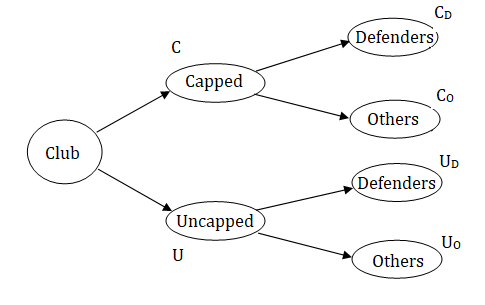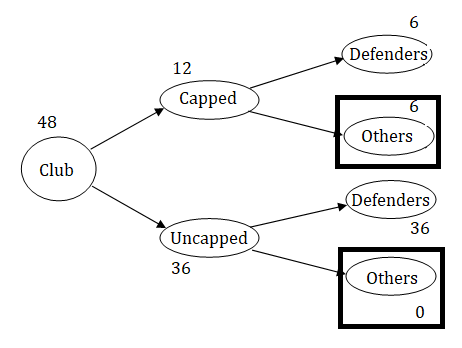GMAT Question of the Day: Daily via email | Daily via Instagram New to GMAT Club? Watch this Video

 It is currently 22 Feb 2020, 14:40### GMAT Club Daily Prep

#### Thank you for using the timer - this advanced tool can estimate your performance and suggest more practice questions. We have subscribed you to Daily Prep Questions via email.

Customized
for You

we will pick new questions that match your level based on your Timer History

Track

every week, we’ll send you an estimated GMAT score based on your performance

Practice
Pays

we will pick new questions that match your level based on your Timer History

#### Not interested in getting valuable practice questions and articles delivered to your email? No problem, unsubscribe here.# In a football club, 1/4th of the players are capped players and ......

Author Message
TAGS:

### Hide Tags

e-GMAT RepresentativeV
Joined: 04 Jan 2015
Posts: 3250
In a football club, 1/4th of the players are capped players and ......  [#permalink]

### Show Tags00:00

Difficulty:15% (low)

Question Stats:88% (01:54) correct12% (02:24) wrongbased on 50 sessions

### HideShow timer Statistics

Everything you need to know about Tree Structures - Exercise Question #2

In a football club, $$\frac{1}{4}^{th}$$ of the players are capped players and the remaining are uncapped players. Half of the capped players and all the uncapped players are defenders. If there are 36 players, in the club, who are uncapped, then what is the number of players, in the club, who are not defenders?

A. 3
B. 6
C. 9
D. 12
E. 18

To read the article: Everything you need to know about Tree Structures

_________________

Originally posted by EgmatQuantExpert on 23 Jan 2019, 03:18.
Last edited by EgmatQuantExpert on 23 Jan 2019, 04:34, edited 1 time in total.
GMAT Club LegendV
Joined: 18 Aug 2017
Posts: 5904
Location: India
Concentration: Sustainability, Marketing
GPA: 4
WE: Marketing (Energy and Utilities)
Re: In a football club, 1/4th of the players are capped players and ......  [#permalink]

### Show Tags

total uncapped players = 36
3*x/4 = 36
x= 48
total players in club = 48
so
capped = 48 * 1/4 = 12

defenders = 6+ 36 = 42

non defenders = 48-42 = 6
IMO B

we can solve question using 2x2 matrix as well..

[
quote="EgmatQuantExpert"]Everything you need to know about Tree Structures - Exercise Question #2

In a football club, $$\frac{1}{4}^{th}$$ of the players are capped players and the remaining are uncapped players. Half of the capped players and all the uncapped players are defenders. If there are 36 players, in the club, who are uncapped, then what is the number of players, in the club, who are not defenders?

A. 3
B. 6
C. 9
D. 12
E. 18

To read the article: Everything you need to know about Tree Structures

[/quote]
e-GMAT RepresentativeV
Joined: 04 Jan 2015
Posts: 3250
In a football club, 1/4th of the players are capped players and ......  [#permalink]

### Show Tags

Solution

Given:
We are given that,
• $$\frac{1}{4}^{th}$$ of the players in a football club are capped players, and the remaining players are uncapped players
o Half of the capped players are defenders

• The number of uncapped players in the club = 36

To find:
• The number of players, in the club, who are not defenders

Approach and Working:
• Total number of players are divided as capped and uncapped, and all the players of these two groups are either defenders are non-defenders.

Now, let’s represent the above structure in the form a tree diagram.Now, let’ see what we need to find : The number of players, in the club, who are not defenders = $$C_O + U_O$$
• Thus, we need to find the values of $$U_O$$ and $$C_O$$, to answer this question

Let us assume that the total number of players in the club = T
• From the given information , we can say that the number of capped players = 25% of T = $$\frac{T}{4}$$
• Which implies, that the number of uncapped players = $$T – \frac{T}{4} = \frac{3T}{4}$$

Now, from the diagram we can see that, total capped players = $$C_D + C_O$$
• Thus, the equation will be $$C_D + C_O = \frac{T}{4}$$
• And, from the given information, we get, $$C_D = (\frac{1}{2}) * (\frac{T}{4}) = \frac{T}{8}$$
o Thus, $$C_O = \frac{T}{4} – \frac{T}{8} = \frac{T}{8}$$ ……………. (1)

We are also given that all uncapped players are defenders
• Thus, $$U_D = \frac{3T}{4}$$ and $$U_O = 0$$ ………………….…… (2)

So, from (1) and (2), we get, $$U_O + C_O = 0 + \frac{T}{8} = \frac{T}{8}$$ ………………. (3)
• To find T, we have information that, number of uncapped players, $$U = \frac{3T}{4} = 36$$
o This implies, $$T = 36 * \frac{4}{3} = 48$$

• Substituting this value of T in (3), we get
o $$U_O + C_O = \frac{48}{8} = 6$$

Therefore, the number of players, in the club, who are not defenders = 6Hence, the correct answer is option B.

_________________
Target Test Prep RepresentativeV
Status: Founder & CEO
Affiliations: Target Test Prep
Joined: 14 Oct 2015
Posts: 9443
Location: United States (CA)
In a football club, 1/4th of the players are capped players and ......  [#permalink]

### Show Tags

EgmatQuantExpert wrote:
Everything you need to know about Tree Structures - Exercise Question #2

In a football club, $$\frac{1}{4}^{th}$$ of the players are capped players and the remaining are uncapped players. Half of the capped players and all the uncapped players are defenders. If there are 36 players, in the club, who are uncapped, then what is the number of players, in the club, who are not defenders?

A. 3
B. 6
C. 9
D. 12
E. 18

We see that the uncapped players comprise 3/4 of the total number of players in the club. Since there are 36 players, the total number of players in the club is 36 / (3/4) = 36 x 4/3 = 12 x 4 = 48. That means 1/4 x 48 = 12 players are capped players. Since 1/2 x 12 = 6 of these 12 capped players (and all the uncapped players) are defenders, the remaining 6 capped players are not defenders.

_________________

# Scott Woodbury-Stewart

Founder and CEO

Scott@TargetTestPrep.com

See why Target Test Prep is the top rated GMAT quant course on GMAT Club. Read Our Reviews

If you find one of my posts helpful, please take a moment to click on the "Kudos" button.In a football club, 1/4th of the players are capped players and ......   [#permalink] 01 Jan 2020, 18:34
Display posts from previous: Sort by

# In a football club, 1/4th of the players are capped players and ......